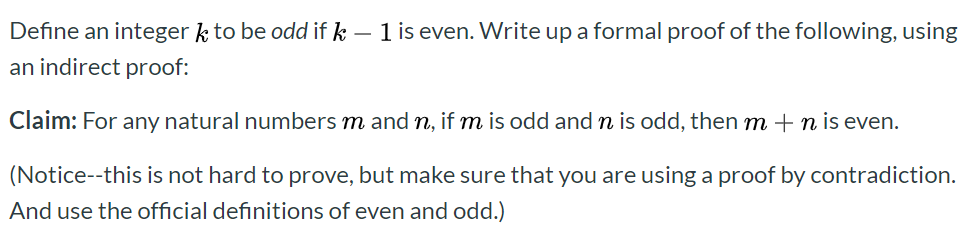# Define an integer k to be odd if k 1 is even. Write up a formal proof of the following, usingan indirect proof:Claim: For any natural numbers m and n, if m is odd and n is odd, then mn is even.(Notice--this is not hard to prove, but make sure that you are using a proof by contradiction.And use the official definitions of even and odd.)

Question
19 views

Define an integer k to be odd if k − 1 is even. Write up a formal proof of the following, using an indirect proof:

Claim: For any natural numbers m and n, if m is odd and n is odd, then m + n is even.help_outlineImage TranscriptioncloseDefine an integer k to be odd if k 1 is even. Write up a formal proof of the following, using an indirect proof: Claim: For any natural numbers m and n, if m is odd and n is odd, then m n is even. (Notice--this is not hard to prove, but make sure that you are using a proof by contradiction. And use the official definitions of even and odd.) fullscreen
check_circle

Step 1

To prove that the sum of any two odd numbers ...

### Want to see the full answer?

See Solution

#### Want to see this answer and more?

Solutions are written by subject experts who are available 24/7. Questions are typically answered within 1 hour.*

See Solution
*Response times may vary by subject and question.
Tagged in

### Math